# 02.03.01: Golden Ratio

## Where to Use

Designs should seek to incorporate aesthetically pleasing proportions into a design.

## Abstraction

Consider using the Golden Ratio and the closely related Fibonacci numbers.

### Golden Ratio

The Golden Ratio is illustrated in the following: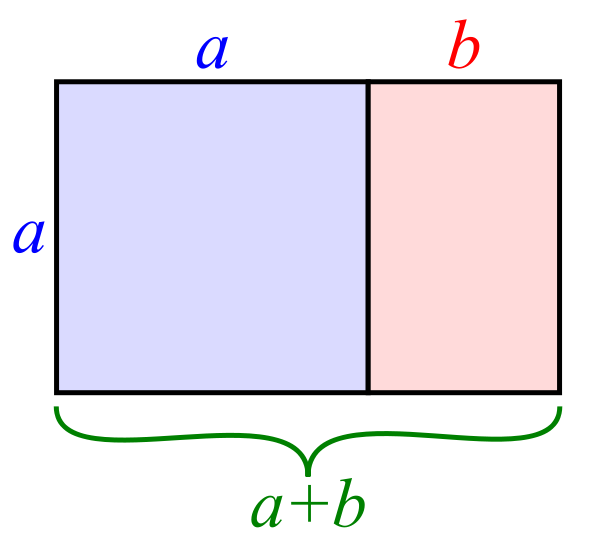Source: Wikipedia
The Golden Ratio is an iterative construction which leads to the following ratios being equal: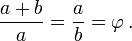This leads to another interesting definition for the Golden Ratio: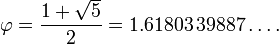The Golden Ratio may also be constructed as follows: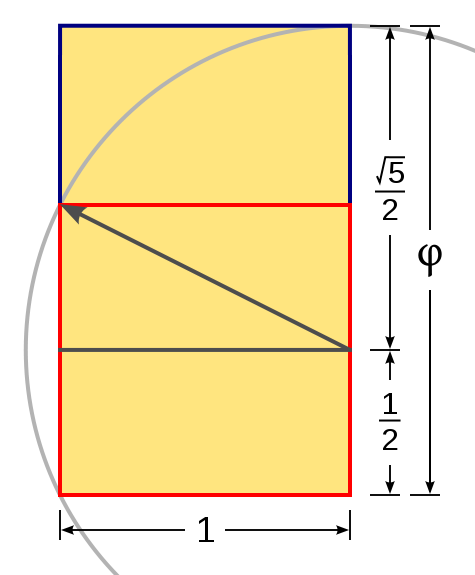### Fibonacci Numbers

The Fibonacci numbers are a sequence created by adding previous numbers to get the next one: 0,1,1,2,3,5,8,13,21… The ratio of a Fibonacci number to the previous one in the series (for example, 13/8) approaches the Golden Ratio as the sequence number increases.

Therefore, consider using dimensions related to Fibonacci numbers (usually through some scaling factor) to create designs that incorporate Golden Ratios and are conveniently related (for example: D:W:H = 5:8:13).

Here is an example using blocks that follow the Fibonacci sequence arrange into the Fibonacci spiral: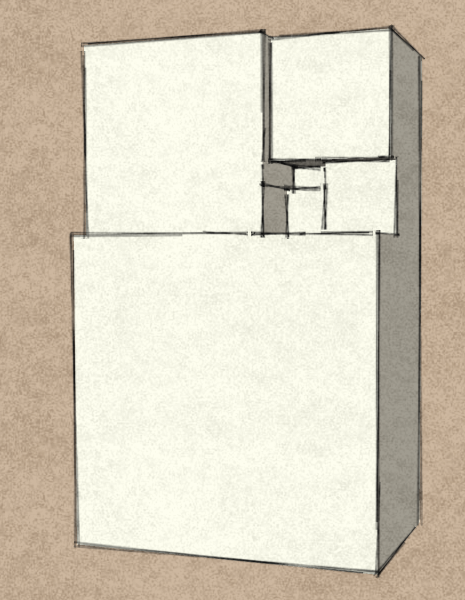## Related Forms

This pattern supports Rabatment.